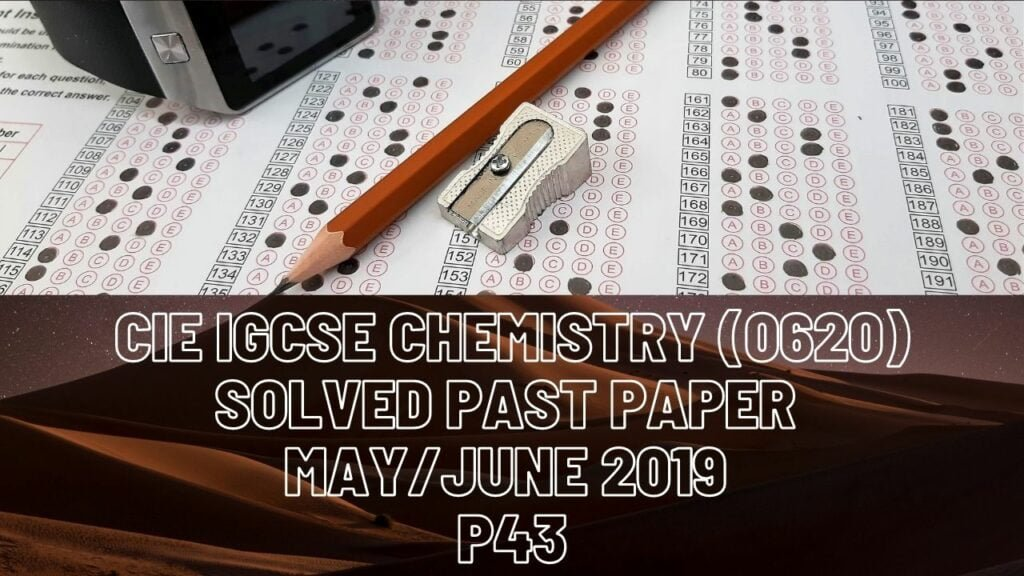IGCSE Chemistry### CIE IGCSE Chemistry Solved Past Paper May/June 2019 P43

1a) Electron   -1

Proton    nucleus

Neutron   1               0

b) Electrons: calcium ion has 2+ charge which means it has two more protons than electrons so electron number = 20-2= 1

Neutrons: mass number – proton number = 44-20 = 24 neutrons

Protons = 20

2a) The number of neutrons

Number of protons

Number of electrons

bi) Chemical reactions depend on the electrons in outer most shell and they all have the same number of electrons in their outer shells

ii) Mg + 2HCl MgCl2 +H2

iii) Lighted splint burns with a squeaky pop

c) Positive ions (atoms that have lost outer shell electrons) are bonded by force of attraction between positive ions and electrons from sea of electrons.

di) Magnesium loses 2 electrons from outer shell to have a complete shell and so gains a charge of 2+. Oxygen gains 2 electrons from magnesium to complete outer shell and gains a charge of 2

ii) due to strong forces of attraction between oppositely charged ions(ionic bonding).

iii) Mg2+ and O2- ions move throughout the structure

3 ai) Low melting point and low density

ii) solid dissolves

b) moles = volume/molar volume = 144/24 = 6 mol

In equation 2NaN3 —- 3N2

2 mol —— 3mol (set ratio)

2 —- 3

X —- 6

x = 6*2/3 = 4 moles of sodium azide Mr = 23 +(14*3) =65

Mass = moles*molar mass

= 4*65 = 260g

c) basic

acidic

di) N3- (as in formula Pb(N3)2 it is shown that 2 molecules of N3 are required to cancel the charge of PB2+ so if 2 ions are required to cancel 2+ charge, that means each ion has charge of 1-)

ii) Pb(NO3)2 + 2NaN3 Pb(N3)2(s) + 2NaNO3(aq)

iii) Filter, and then wash with water

e) Start by writing symbols of compound: C:H:N

Then calculate their moles

49.5/12–7.2/1– 43.3/14 = 4.125      7.2      3.093

Now calculate ratio by dividing all values with least value (3.093)

= (1.33     2.33      1) multiple by 3 to obtain whole number value

= 4:7:3——> C4H7N3

4 ai) It is inert

ii) Green coloured gas bubbles form

iii) Mass would increase due to copper metal formation on its surface

iv) Turns colourless, because cu ions are no longer in ions form

v) Chloride ion, because it loses electron

bi) Anode as silver (it oxides losing electron here). Cathode as spoon and solution of aqueous silver nitrate.

ii) Better appearance

5 ai) Ethyl ethanoate

ii) Ester group on second carbon on chain

b) Products have less energy than the reactants

c) It increases rate of reaction of a chemical reaction without being used up

d) Molecules gain kinetic energy and move faster so collision rate increases which means more molecules have sufficient energy to react.

ei) Amount decreases because backwards reaction is endothermic so equilibrium shifts left.

ii) More amount as equilibrium shifts to right to replace water

6 ai) Compounds with carbon and hydrogen atoms only

ii) Bromine water turns colourless, orange -brown